# AP Calculus BC Practice Test 20

### Test Information8 questions24 minutes

Calculator Allowed

1. The coefficient of x3 in the Taylor series of ln (1 - x) about x = 0 (the Maclaurin series) is

2. The rate at which a rumor spreads across a campus of college students is given by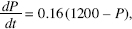where P(T) represents the number of students who have heard the rumor after t days. If 200 students heard the rumor today (t = 0), how many will have heard it by midnight the day after tomorrow (t = 2)?

3.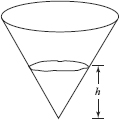Water is poured at a constant rate into the conical reservoir shown above. If thedepth of the water, h, is graphed as a function of time, the graph is

4. A 26-foot ladder leans against a building so that its foot moves away from the buildingat the rate of 3 feet per second. When the foot of the ladder is 10 feet from thebuilding, the top is moving down at the rate of r feet per second, where r is

5. If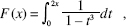then F (x) =

6.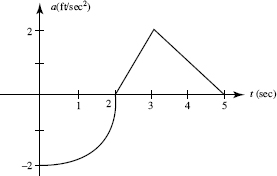The graph above shows an object's acceleration (in ft/sec2). It consists of a quarter-circle and two line segments. If the object was at restat t = 5 seconds, what was its initial velocity?

7. Water is leaking from a tank at the rate of R(t) = 5 arctan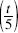gallons per hour, where t is the number of hours since the leak began. How many gallons will leak out duringthe first day?

8. The first-quandrant area inside the rose r = 3 sin 2θ is approximately